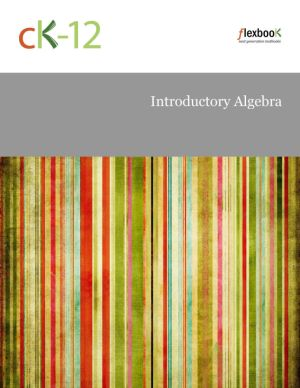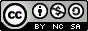# Introductory Algebra

by Anne Gloag, Andrew Gloag, Melissa KramerJoin

### Book Description

In order to represent real life situations mathematically, we often use symbols to represent unknown quantities. We call these symbols variables. Each mathematical subject requires knowledge of manipulating expressions and equations to solve for a variable. Careers such as automobile accident investigators, quality control engineers, and insurance originators use equations to determine the value of variables. Real numbers are all around us.

Introductory Algebra provides a comprehensive introduction of variables, functionsand linear equations and gives students a solid foundation in algebra.

Chapter 1
Arithmetic Review
Chapter 2
Introduction to Variables
Chapter 3
Polynomials and Exponents, Part 1
Chapter 4
Polynomials and Exponents, Part 2
Chapter 5
Solving Equations
Chapter 6
Linear Equations: Real World Applications
Chapter 7
Literal Equations and Inequalities
Chapter 8
Graphs and Graphing Linear Equations
Chapter 9
Introduction to Functions
Chapter 10
Linear Functions
Chapter 11
More on Linear Functions
Chapter 12
Systems of Linear Equations
Chapter 13
Problem Solving

### Book Details

Title
Introductory Algebra
Subject
Science and Mathematics
Publisher
Independent
Published
2013
Pages
297
Edition
2
Language
English
ISBN13 Digital
9781607826590
ISBN10 Digital
1607826593
PDF Size
38.6 MB### Related BooksThis book studies the foundations of quantum theory through its relationship to classical physics. This idea goes back to the Copenhagen Interpretation (in the original version due to Bohr and Heisenberg), which the author relates to the mathematical formalism of operator algebras originally created by von Neumann. The book therefore includes compr...This carefully written textbook offers a thorough introduction to abstract algebra, covering the fundamentals of groups, rings and fields. The first two chapters present preliminary topics such as properties of the integers and equivalence relations. The author then explores the first major algebraic structure, the group, progressing as far as the ...This survey of the state of the art on research in early algebra traces the evolution of a relatively new field of research and teaching practice. With its focus on the younger student, aged from about 6 years up to 12 years, this volume reveals the nature of the research that has been carried out in early algebra and how it has shaped the growth o...This book develops the essential tools of linear algebra, with the goal of imparting technique alongside contextual understanding. Applications go hand-in-hand with theory, each reinforcing and explaining the other. This approach encourages students to develop not only the technical proficiency needed to go on to further study, but an appreciation ...Linear Algebra with Applications by W. Keith Nicholson, traditionally published for many years is now being released as an open educational resource. Overall, the aim of the book is to achieve a balance among computational skills, theory, and applications of linear algebra. It is a relatively advanced introduction to the ideas and techniques of ...Introductory Statistics follows scope and sequence requirements of a one-semester introduction to statistics course and is geared toward students majoring in fields other than math or engineering. The text assumes some knowledge of intermediate algebra and focuses on statistics application over theory. Introductory Statistics includes innovative pr...